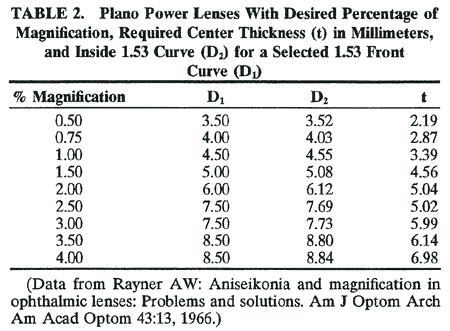THRESHOLDS OF ANISEIKONIA INCIDENCE OF ANISEIKONIA EFFECTS ON VISUAL FUNCTIONING AXIAL VERSUS REFRACTIVE ANISOMETROPIA RETINAL IMAGE SIZE ESTIMATING THE AMOUNT OF ANISEIKONIA PRESCRIBING ANISEIKONIA LENSES CALCULATING THE CURVES USING THE THILL ANISEIKONIA WORKSHEET SUMMARY REFERENCES
Aniseikonia, a term coined by Dr Walter Lancaster in 1932, is an anomaly of binocular vision in which the ocular images are unequal in size, shape, or both. (The ocular image is the final impression received in the higher cortical centers, involving the retinal image with modifications imposed by anatomic, physiologic, and perhaps psychological properties of the entire binocular visual apparatus.) In general, aniseikonia is associated with a false stereoscopic localization and an apparent distortion of objects in space.1 Aniseikonia can be the cause of asthenopia, diplopia, suppression, poor fusion, headaches, vertigo, photophobia, amblyopia, and strabismus.

The differences in size may be overall—that is, the same in all meridians—or meridional, in which the difference is greatest in one meridian and least in the meridian 90 degrees away. The effect of aniseikonia on space perception is usually dramatic, especially when meridional magnification is involved. This is particularly true when the horizontal meridian is involved, since horizontal disparities are the basis for the stereoscopic perception of depth.1

Aniseikonia was thoroughly researched at Dartmouth College in Hanover, NH, from 1920 to 1945 under the leadership of Adelbert Ames. Brought together at the time were some of the leading scientists of the day, including Hermann M. Burian, Paul Boeder, Robert Bannon, Walter B. Lancaster, Arthur Linksz, and Kenneth N. Ogle. Although their studies were involved with all binocular anomalies, they were particularly interested in aniseikonia.

The most obvious cases of aniseikonia, those with anisometropia, are discussed here, but one must bear in mind that there are many cases on record of patients with emmetropia or isometropia who had aniseikonia that, when corrected, relieved that patient of symptoms. These cases are further proof that aspects other than retinal image size are involved in aniseikonia. Factors such as retinal element distribution, differences in number of radiation fibers, possible modification at the synapses, and indeed the physiology of the entire neurovisual system play a part in the final perceived image size.

One study reported objective evidence of the benefits of aniseikonic correction.2 An immediate reproducible increase in amplitude of both steady-state and transient binocular visual evoked responses (VERs) was demonstrated when aniseikonic correction was included in a patient's spectacle correction. In addition, after this patient wore the aniseikonic correction for 3 months, she reported a lessening of subjective complaints. There was great improvement in visual comfort, decreased frequency of headaches, and an increased ability to read for extended periods. The case history also suggested that the frequency of her intermittent exotropia had decreased. Additionally, there was now no indication of suppression at the patient's normal reading distance, and stereoacuity had increased from 100 to 30 seconds of arc.

 THRESHOLDS OF ANISEIKONIA Tolerances for aniseikonia vary from patient to patient; some apparently are able to tolerate rather large amounts, and others have severe symptoms with much smaller levels. It is when the difference in image size or meridional distortions approaches the patient's tolerance that the symptoms of aniseikonia become manifest and troublesome. We may define clinical aniseikonia as the amount of aniseikonia that must be corrected to eliminate the patient's symptoms.Clinical aniseikonia usually occurs when the difference in image size between the two eyes approaches 0.75%. Meridional distortions are more poorly tolerated, especially when they are oblique (not horizontal or vertical). Oblique meridional aniseikonia causes a rotary deviation between the fused images of vertical lines in the two eyes; this is termed declination. Declination becomes clinically troublesome when it approaches 0.3 degrees.
 INCIDENCE OF ANISEIKONIA The estimation of the incidence of aniseikonia varies with the investigator and with the population studied. According to the Dartmouth College study, which spanned 15 years, aniseikonic lenses were prescribed for 4% of the population of Hanover. In their follow-up study, the researchers found that about 75% of these patients declared they had benefited from the correction, which would indicate that 3% of the general population need and benefit from the correction of an aniseikonia.Gillott, in England, examined a cross section of a community and found 8% to have a significant aniseikonia, associated with symptoms.3 Ewalt, who used eikonometry for a lengthy period, suggested the clinically significant incidence to be about 2%.4 Duke-Elder said that some degree should be expected in 20% to 30% of people who wear spectacle correction.5A study of different groups shows differences in occurrence. Burian and Triller showed that the composition of the group influences the incidence (Table 1)6.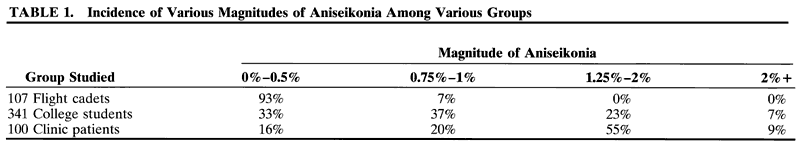EFFECTS ON VISUAL FUNCTIONING Different authors show a similarity in the nature of symptoms and their frequencies.4,7 Symptoms, in order of their frequency of occurrence, are local eye discomfort (tiring), headaches, difficulty reading, photophobia, poor depth perception, vertigo, nausea, motility difficulty, nervousness, and physical fatigue.
 AXIAL VERSUS REFRACTIVE ANISOMETROPIA Although aniseikonia can be measured using an instrument such as the American Optical Space Eikonometer, these instruments are not widely available. Remole and Robertson list several direct comparison tests using equipment usually found in the refracting room, such as Brecker's device.8 Another direct comparison test, the Awaya NAT (New Aniseikonia Test) is available from Binocular Vision Quarterly.*9 It consists of a booklet containing a series of red and green half moons (dissociated with red and green spectacles) differing in size from 1% to 24%.8We suspect aniseikonia may be present in cases of anisometropia. Anisometropia may be divided into two major categories: axial, in which the difference between the two eyes is due to a difference in their axial lengths, and refractive, in which the ametropic difference between the two eyes occurs as a result of differences in corneal or lens power, or position of crystallin lens.Clinical rules for applying these concepts are as follows:If the anisometropia is 2 D or more, assume it is axial.10If the anisometropia is less than 2 D or is in cylinder only, assume it is refractive. Astigmatism is classified as a cause of refractive aniseikonia if it is found in only one eye, if the power needed is greater in one eye than the other, or if the axes in each eye differ from one another.
 RETINAL IMAGE SIZE IN UNCORRECTED REFRACTIVE AMETROPIAIf we start with a hypothetical emmetropic eye and make it ametropic by changing only the power of its cornea or lens (refractive ametropia), this uncorrected eye now receives a blurred image. The size of this uncorrected image is changed only minimally, the myopic eye having a slightly smaller image by 0.25% per diopter and the hyperopic eye having a slightly larger image by 0.25% per diopter.IN CORRECTED REFRACTIVE AMETROPIACorrecting refractive ametropia with spectacles placed at the anterior focal point (about 15 mm from the cornea) causes the resultant image to be clear but now different in size from the emmetropic eye by 1.5% per diopter, smaller in myopia and larger in hyperopia. Placing the lens closer to the cornea than 15 mm will result in less minification for the minus lens or less magnification for the plus lens. Placing the refractive correction on the cornea (contact lenses) gives the least change in image size from the uncorrected state in refractive ametropia. If contact lenses can be tolerated and the ametropia is of refractive nature, it is possible that no other correction for aniseikonia may be necessary.IN UNCORRECTED AXIAL AMETROPIALet us consider the case of our hypothetical emme-tropic eye, this time made ametropic by varying only the axial length. This eye then receives a blurred image but with a significant change in image size as well. The axially myopic eye has a larger image by about 1.50% per diopter and the axially hyperopic eye has a smaller image by 1.5% per diopter.IN CORRECTED AXIAL AMETROPIAPlacing the correcting lens at the anterior focal point of the eye (about 15 mm from the cornea) again causes a 1.5% per diopter change in size of the image from what it was when uncorrected, just as in the previously discussed refractive case, with the minus lens decreasing the size by 1.5% per diopter and the plus lens increasing the size by 1.5% per diopter. However, the uncorrected axial image size as stated above is larger in myopia and smaller in hyperopia. (Knapp's law: in the axially ametropic eye, if the correcting lens is placed before the eye so that the second principal plane of the lens coincides with the anterior focal point of the eye, the size of the image on the retina will be the same as if the eye were emmetropic11).Theoretically, although axial ametropes would not need aniseikonic correction if the correcting lenses were placed at the anterior focal point, these patients often can be made far more comfortable by judicious use of the techniques we will discuss. Studies show that sometimes minification is needed for axially myopic eyes and magnification is needed for axially hyperopic eyes,12–16 just as in the case of the refractive ametrope.The reasoning behind this is as follows: whereas the retinal image of the corrected eye may be the same as in emmetropia as stated in Knapp's law, the final image received in the higher cortical centers is different because the retinal elements have been either scattered or condensed through stretching or shrinking of the retina associated with the change in axial length. For example, when the axial length of the eye increases, the retina must also stretch so that the retinal element population is decreased for a given area, thereby causing the patient to perceive a smaller image since fewer retinal elements are being stimulated. The opposite occurs for hyperopia caused by an axially shortened eye.
 ESTIMATING THE AMOUNT OF ANISEIKONIA The guidelines given by the Dartmouth studies for estimating the amount of aniseikonia were as follows: If the difference in image size associated with anisometropia is primarily of refractive origin, we can expect it to be about 1.5% per diopter of anisometropia, but since the anisometropia may be partly axial, an estimate of 1% per diopter is more useful clinically. The eye requiring the more myopic, or less hyperopic, correction would be expected to require the magnification.
 PRESCRIBING ANISEIKONIA LENSES When deciding which spectacle lens to modify it is important to realize that the size and shape of the final image does not matter; it is only important that the images of each eye match each other. In designing lenses, instead of magnifying the image of one eye it may be easier to minify the image in the other eye. In doing so, it may be possible to thus present to the patient a more cosmetically acceptable pair of spectacles, or perhaps lenses that are more easily manufactured and therefore less costly.The total magnification of a lens (Mt), in percent, is found by adding the magnification in percent from its power (Mp) and the magnification in percent from its shape (Ms):(eq 1)Mt = Mp + MsMagnification from power (Mp) is dependent on the dioptric power of the lens (Dv) and its vertex distance (h). If h is measured in millimeters the relationship is(eq 2)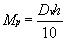Based on the relationship given by equation 2, if Dv (the power of the lens) is positive (a plus lens), the magnification will be positive, but if Dv is negative (a minus lens) the magnification will be negative—that is, a minification.If h, the vertex distance, is changed, the following are true:Moving a lens away from the eye (increasing the vertex distance) increases the magnification of a plus lens and the minification of a minus lens.Moving a lens toward the eye (decreasing the vertex distance) decreases the magnification of a plus lens and the minification of a minus lens.Magnification from shape (Ms) depends on the curvature of the front surface of the lens (D1) and the center thickness of the lens (t). The 1.5 in the following equation is the index of refraction (approximately) of glass or plastic.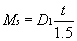In this form, the center thickness is in centimeters. To use millimeters, divide by 10 and the formula becomes(eq 3)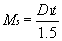The more curved the lens (the larger the D1), the more magnification from shape and the thicker the lens (t), the more magnification from shape.However, a change in either the front curve or the thickness of the lens will also cause the vertex distance, h, to be changed so that the magnification from the power factor (Mp) is also affected.If the front curve is changed to give the magnification or minification needed, the back curve must also be changed to maintain the same power of the lens. Therefore, if, for example, the front curve is increased, the back curve must also be increased, which increases the vertex distance. If the front curve is flattened, the back curve must also be flattened, which causes the vertex distance to be decreased.If center thickness is increased to increase the magnification of the lens but the front curve is left the same, the increase moves the back surface closer to the eye by the amount of the increase, thereby decreasing the vertex distance. On the other hand, if the center thickness is decreased but the front curve is left the same, the decrease causes the vertex distance to be increased by that amount.Clinically it is rare to have to correct an aniseikonia of larger than 4% to 5%. Patients with greater degrees of aniseikonia have such a large disparity in image size that they generally do not have binocularity.It is usually assumed that patients can comfortably tolerate up to 1% of aniseikonia in nonastigmatic cases.
CALCULATING THE CURVES USING THE THILL ANISEIKONIA WORKSHEET

BASIC CALCULATION

The Thill Aniseikonia Worksheet is shown in Figure 1.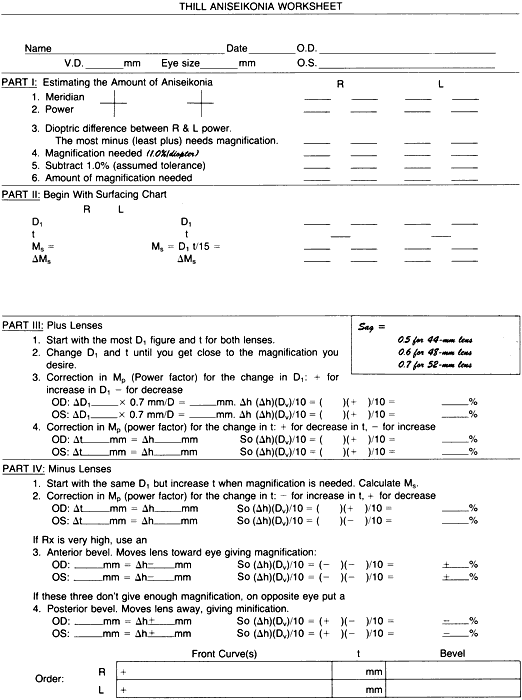Fig. 1. Thill Aniseikonia Worksheet.

Part I

Each meridian must be compared with its opposite in the other eye, so it is helpful to begin by putting the powers on an optical cross. (Most axes are close to the vertical or horizontal.)

Line 1: Using the patient's prescription, indicate the meridian closest to 180 and to 90 degrees.
Line 2: Fill in the power in that meridian.
Line 3: The meridian that needs the magnification. Calculate the difference between the two 180-degree meridians and put this difference in the column that has the most minus or the least plus in the prescription. Do the same for the 90-degree meridian. If the axes are at 45 and 135 degrees, use those meridians instead.
Line 4: Gross magnification required. The figure from line 3 is multiplied by 1% per diopter. If some test was used for aniseikonia and a percentage was found, skip lines 1, 2, and 3; use the test figure on line 4.
Line 5: Subtract 1%, which it is assumed the patient can tolerate.
Line 6: Gives the amount of magnification we will attempt to create.

Part II

Using a surfacing chart and using minus cylinders to get a starting point, find the front curve D1 and the center thickness with which the lens would ordinarily be fabricated. Calculate the magnification from shape using this information, and enter it into the space to the left:

(eq 4)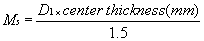Part III

Line 1: Use the figure with the higher front curve and center thickness for both lenses. Enter this information in pencil (so that it can be modified if necessary) in the correct lines to the right. Calculate Ms using the formula in equation 4.
Line 2: Experiment with how these figures should be changed to obtain the desired magnification or minification required from line 6. Begin by decreasing the curve on the lens that requires the minification. Calculate Ms using this new, flatter curve.
Line 3: When magnification is close to that desired, a correction must be made for the change in magnification due to the power factor, Mp, caused by this change in the front curve, D1. To do this we must know the change in the vertex distance created by the change in D1. There is a relationship between curve and width of the lens, called sag:d

0.5 mm per diopter for a 44-mm lens
0.6 mm per diopter for a 48-mm lens
0.7 mm per diopter for a 52-mm lens

This means, for example, that for a 44-mm lens, if the front curve has been changed by 1 D, the vertex distance changes by 0.5 mm.dFor a plus lens:

 if (D1) is Thickness is Vertex distance is Resulting in Increased Increased More Magnification Decreased Decreased Less Magnification

Add this correction to the Ms found in line 2. If this does not give the required minification, decrease center thickness slightly and recalculate Ms.

Line 4: Correction in Mp (power factor) for the change in center thickness. Again, owing to change in vertex distance, now due to the change in center thickness, another correction in Mp is to be made. For plus lenses:

 If Center Thickness is Vertex distance is Resulting in Decreased Increased More Magnification Increased Decreased Less Magnification

Add this correction to the Ms found in Part II.

If the magnification is still not the desired amount, go to the other lens to increase the front curve and increase the center thickness, correcting both changes for Mp changes as was done in the above (Fig. 2).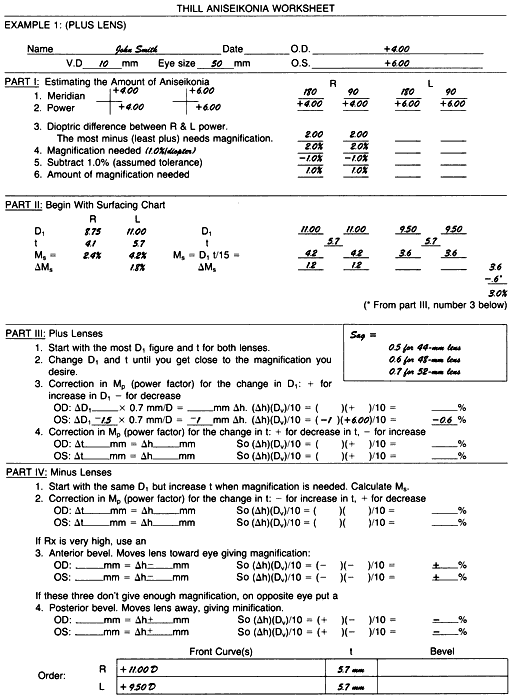Fig. 2. Example 1: a plus lens problem.

Part IV

For explanation, see later discussion Example 2, Minus-Lens Problem.

EXAMPLE 1: A PLUS LENS PROBLEM

The right eye requires a + 4.00-D lens and the left eye a + 6.00-D lens. Vertex distance, h, is 10 mm, and the lens will be 50 mm wide (see Fig. 2).

Part I

The + 4.00 D is put on the optical cross for both horizontal and vertical meridians for the right eye, and the + 6.00 D is put on the optical cross for both horizontal and vertical meridians for the left eye.

Line 1: Since lenses are spheres, all the meridians are the same.
Line 2: Power will be + 4.00 D for both meridians on the right eye and + 6.00 D for both meridians on the left eye.
Line 3: Difference is 2.00 D for both horizontal and vertical meridians and is placed in the columns that have the least plus, the right eye in this case.
Line 4: Magnification estimation is 1% per diopter, yielding 1% × 2.00 D = 2%.
Line 5: Subtracting the 1% assumed tolerance.
Line 6: Gives the amount of magnification desired as 1% in both meridians of the right eye, an “overall” correction.

Part II

The surfacing chart gives 8.75 D for D1 and a4.1-mm center thickness for the right lens and11.00 D for D1 and a 5.7-mm center thickness for the left lens. Calculating the Ms from shape gives us the amount of magnification we would receive from the lenses if the lenses were ordered as “stock” lenses:

Ms = 8.75 × 4.1/15 = 2.4% for the right eye

Ms = 11.00 × 5.7/15 = 4.2% for the left eye

These lenses would give us a net 1.8% magnification on the left eye, not at all what was required, which was 1% magnification on the right eye.

Using the larger D1 and center thickness figure, place these amounts in the lines to the right of Part II. (Using the identical curves and center thicknesses on each lens would give zero magnification difference from Ms factor, which, while it is better than the stock lenses, is still not the 1% magnification required for the right eye.)

Decrease the front curve on the left lens to9.5 D. Recalculate Ms:

Ms = 9.5 × 5.7/15 = 3.6%

Part III

Correct the Mp. For this change of D1, enter on line 3 the 1.5-D difference:(This is a minification, since it represents a decrease in vertex distance.) The magnification from Ms with this change in D1 is then 3.6% - 0.6% = 3.0%.

The right lens is 4.2% and the left lens is now 3%, and the difference is 1.2%, which is very close to the 1% needed. There is no need to change t, center thickness. This is a practical endpoint.

Discussion

To decide which curve to use on the left lens for the front surface, I first consulted the surfacing chart to see what curves are available that are less than the 11.00-D curve on the right lens. I found these three possible curves: 10.25, 9.50, and 8.75 D.

The 10.25 D gives shape magnification of 10.25 × 5.7/15 = 3.9%, which did not seem to be enoughof a change, whereas the 8.75-D curve was 8.75 × 5.7/15 = 3.3%. At first this seemed to be the curve of choice, but when the power factor was calculated, the difference, (11.00 - 8.75) = 2.25 D, gave a1.6-mm vertex distance change (2.25 × 0.7), and this vertex distance change gives minification of 1.0%: (1.6)(+ 6.00)/10 = -1%.

This 1% loss of magnification when added to the 3.3% would have resulted in 2.3% for the left lens, and this when subtracted from 4.2% of the right lens would become 1.9% magnification on the right eye, which is 0.9% too much magnification.

Recalculating, using the 9.5-D figure gave the correct answer. Other possibilities include the following:

• The curve on the right lens could have been increased to give the 1% magnification needed for the right eye, but 11.00 D is already a very strongly curved lens and any more added to it would make it appear strange. Had the problem required more magnification than the 1%, this would have been a feasible alternative, however.
• Increasing the center thickness on the right eye would also have been a good suggestion, but increasing the center thickness would decrease the vertex distance, which in turn would have caused a loss of magnification from the power factor. It would also increase the weight of the lens.
• With regard to the left lens, we could have decreased the center thickness to attain less magnification, but again this would have been offset by an increase in vertex distance, which would resultin more magnification. Also, in the case of a thinner lens, care must be taken that the edge thickness is not too thin or there will be danger of the lens chipping, or the laboratory may even reject the instructions as being impractical. For this reason it is wise to try to keep the lens size as small as possible by encouraging the patient to select a frame with a relatively small eye size.

EXAMPLE 2: MINUS LENS PROBLEM

The right eye requires a -1.00-D lens and the left eye a -3.00-D lens. Vertex distance, h, is 15 mm, and the lens will be 48 mm wide (Fig. 3).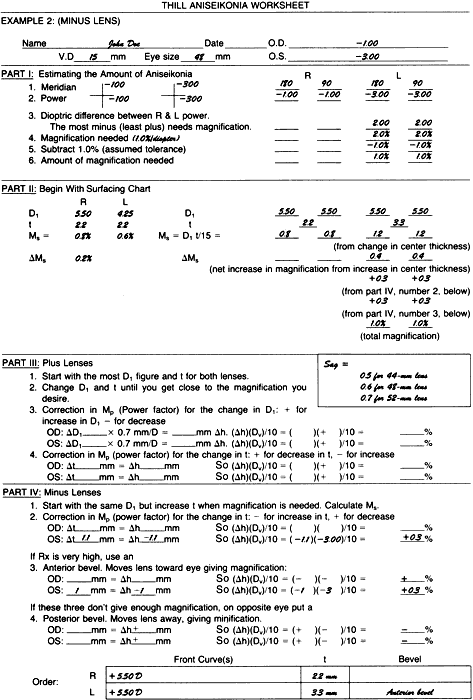Fig. 3. Example 2: a minus lens problem.

Part I

Using the same directions given in the plus lens problem of Figure 2, fill out Part I of the Thill Aniseikonia Worksheet down to line 6, the amount of magnification needed, which in this example is 1% on the left eye.

Part II

The surfacing chart indicates that at a 5.50-D curve would be used on the right lens and a 4.25-D curve on the left lens, with both center thicknesses being 2.2 mm. Calculating the Ms from shape gives us the amount of magnification we would receive from the lenses if the stock lenses were ordered.

Ms = 5.5 × 2.2/15 = 0.8% for the right eye

Ms = 4.25 × 2.2/15 = 0.6% for the left eye

Part IV

Dropping down to the instructions for minus lenses, line 1 states to begin with the same D1 for the front curves of both lenses and increase center thickness where magnification is needed. Begin by first making both lenses the same:

 R L D1 5.50 5.50 t 2.2 2.2 Ms 0.8% 0.8%

and increase the center thickness on the left lens where the magnification is required. If we increase to 3.3-mm center thickness, Ms becomes 5.5 × 3.3/15 = 1.2%. Comparing this with the right lens, we now have 0.4% magnification on the left eye. We need 0.6% more. However, first note the change in Mp (power factor).

Line 2 is the correction in Mp for the change in t:The increase in center thickness resulted in a decrease in vertex distance, which in turn resulted in an increase in magnification (decrease in minification) of 0.3%. This 0.3% when added to the 1.2% gives a total magnification on the left eye of 1.5%. Subtracting the 0.8% magnification of the right lens leaves a difference of 0.7%. This is an improvement, but we still need 0.3% more magnification on the left eye.

Part IV

With an anterior bevel, we can move the lens toward the eye, giving magnification (decreased minification). Request that the left lens be beveled to the extreme anterior edge, which for a -3.00-D power lens and a 48-mm diameter will have an edge thickness between 4 and 5 mm. If we consider that this will amount to 1 mm: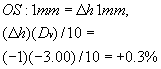This 0.3% when added to the 1.5% equals 1.8%. Thus, the left lens now has a magnification of 1.8%, which, when compared with the 0.8% magnification of the right lens gives us the required net magnification of 1% in the left lens.

The order to the laboratory would be as follows:

Rt lens: 5.50 D front curve; 2.2 mm t Lt lens: 5.50 D front curve; 3.3 mm t, anterior bevel

Discussion

The right lens could have been decreased in its magnification. However, this would be difficult since the power is low and the center thickness is at the legal minimum limit.

For example, if we tried to decrease the magnification of the right lens by decreasing the front curve to 3.25 D:

Ms = 3.25 × 2.2/15 = 0.5%

or a decrease of 0.3%. This would be offset by the power factor change of 5.50 - 3.25 = 2.25 D.

And, 2.25 × 0.6 (for a 48-mm lens) = 1 mmthe lens moves closer to the eye, and 1 mm(-1.00 D)/10 = 0.1% decrease in minification (increase in magnification). So 0.5% plus 0.1% = 0.6% total magnification from shape.

Comparing this with the previous 0.8% shape magnification, we see that decreasing the front curve to decrease the magnification resulted only in a difference of 0.2% (0.8% - 0.6%).

If we tried to increase the magnification of the left eye by increasing the front curve (instead of increasing the center thickness as the example showed), presume a 7.25-D front curve:

Ms = 7.25 × 2.2/15 = 1.06%

which does give about a 0.2% increase in magnification from shape, but we lose 0.3% when we correct for power factor change:Since this represents a movement away from the eye, the result is a minification. Hence, the 1.06% figure must be decreased by this 0.3%, which results in about 0.7%. This is slightly less magnification than the 0.8% we had with the original 5.50-D and2.2-mm center thickness lens.

Rule: On minus lenses, if the lens power is over -2.00 D, it is not possible to get a net increase in magnification by changing the front curve. Some magnification is achieved from the shape factor, but the power factor has a greater influence, so the total effect of the two is a minification.

Minus lenses thus present a special problem when trying to gain magnification. The best solution is to work with the center thickness, increasing this where magnification is needed, since this will give magnification from both the shape factor, Ms, and the power factor, Mp.

Next, if still more magnification on a minus lens is needed, decrease the front curve on the eye needing the magnification. This further decreases the vertex distance and adds to the magnification (decreases minification). There will also be a slight change from the shape factor with the flatter lens, but the power factor has more influence.

Third, the bevel can be put on to the extreme front of the lens so that the vertex distance is reduced as much as possible. Minus lenses, having large edges, can be manipulated quite easily in this manner.

If these three changes do not give the magnification needed, the lens of the opposite eye (assuming it is also a minus lens) can be beveled to the back to increase the vertex distance, thereby minifying that image.

EXAMPLE 3: A CASE OF ASTIGMATISM

The right eye requires + 1.00 – 3.00 × 180 and the left eye requires plano -1.00 × 180. Vertex distance is 10 mm.

Part I

Put the powers on the optical cross, being certain they are in the correct meridian. From the optical cross, transfer the powers to the correct meridian in the columns. In this case, there is more minus in the right eye in the vertical meridian and more minus (less plus) in the left eye in the horizontal meridian.

Magnification needed is 1% per diopter. There will be no allowance made for tolerance, since this is astigmatic and tolerance is less than in purely spherical cases.

Part I should appear as shown in Figure 4.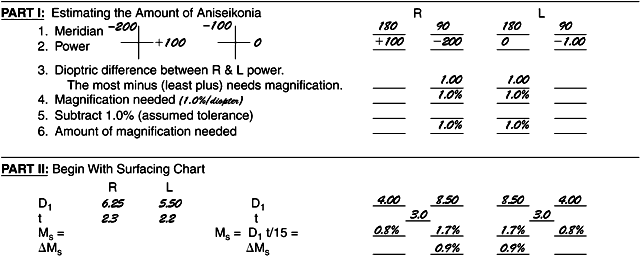Fig. 4. Example 3: a case of astigmatism.

Begin with the surfacing chart. The lenses for these powers would be made up with a curve on the right eye of 6.25 D and for the left eye of5.50 D. Center thickness would be 2.3 mm for the right lens and 2.2 for the left lens. Using this information as a starting point, flatten the curves for the meridians where the least magnification is needed and increase the curves for the meridians where the most magnification is needed. There will therefore be two curves on the front of each lens. Keep the center thicknesses the same and manipulate only the front curves.

Part II will look something like shown in Figure 4. These lenses would be called bitoric because they have toric curves on both the front and the back surfaces.

TRIAL ANISEIKONIC LENSES IN CLIP-ONS

One way to determine if there is an aniseikonic condition without going to the expense of first ordering aniseikonic lenses would be to use special clip-on lenses that have magnification but no power. The configuration for these lenses might be as shown in Table 2, although there could be other combinations to attain the same results.17